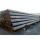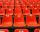# Loan 5

Abdul takes a loan of 200000 from Ali and agrees to repay in number of instalment, each instalment begin with the 2nd exceeding the previous one by 1000, if the first instalment is 500, find how many instalment will be necessary to be wipe out
the loan? Completely

Result

n =  20

#### Solution:

$a = 200000 \ \\ a_{ 1 } = 500 \ \\ d = 1000 \ \\ \ \\ a = \dfrac{ a_{ 1 }+a_n }{ 2 } n \ \\ a = \dfrac{ a_{ 1 }+a_{ 1 }+(n-1) \cdot \ d }{ 2 } \cdot \ n \ \\ \ \\ \ \\ 200000 \cdot \ 2 = (500 \cdot \ 2 + (n-1) \cdot \ 1000) \cdot \ n \ \\ -1000n^2 +400000 = 0 \ \\ 1000n^2 -400000 = 0 \ \\ \ \\ a = 1000; b = 0; c = -400000 \ \\ D = b^2 - 4ac = 0^2 - 4\cdot 1000 \cdot (-400000) = 1600000000 \ \\ D>0 \ \\ \ \\ n_{1,2} = \dfrac{ -b \pm \sqrt{ D } }{ 2a } = \dfrac{ \pm \sqrt{ 1600000000 } }{ 2000 } \ \\ n_{1,2} = \dfrac{ \pm 40000 }{ 2000 } \ \\ n_{1,2} = \pm 20 \ \\ n_{1} = 20 \ \\ n_{2} = -20 \ \\ \ \\ \text{ Factored form of the equation: } \ \\ 1000 (n -20) (n +20) = 0 \ \\ n = n_{ 1 } = 20$

Checkout calculation with our calculator of quadratic equations.

Leave us a comment of this math problem and its solution (i.e. if it is still somewhat unclear...):Be the first to comment!#### Following knowledge from mathematics are needed to solve this word math problem:

Looking for help with calculating roots of a quadratic equation? Do you have a linear equation or system of equations and looking for its solution? Or do you have quadratic equation?

## Next similar math problems:

1. Theorem proveWe want to prove the sentence: If the natural number n is divisible by six, then n is divisible by three. From what assumption we started?
2. SequenceBetween numbers 1 and 53 insert n members of the arithmetic sequence that its sum is 702.
3. The sumThe sum of the first 10 members of the arithmetic sequence is 120. What will be the sum if the difference is reduced by 2?
4. Cinema 4In cinema are 1656 seats and in the last row are 105 seats , in each next row 3 seats less. How many are the total rows in cinema?
5. Solve 3Solve quadratic equation: (6n+1) (4n-1) = 3n2
6. EquationEquation ? has one root x1 = 8. Determine the coefficient b and the second root x2.
7. AS sequenceIn an arithmetic sequence is given the difference d = -3 and a71 = 455. a) Determine the value of a62 b) Determine the sum of 71 members.
8. RootsDetermine the quadratic equation absolute coefficient q, that the equation has a real double root and the root x calculate: ?
9. Six termsFind the first six terms of the sequence a1 = -3, an = 2 * an-1
10. TubesIron tubes in the warehouse are stored in layers so that each tube top layer fit into the gaps of the lower layer. How many layers are needed to deposit 100 tubes if top layer has 9 tubes? How many tubes are in bottom layer of tubes?
11. SeatsSeats in the sport hall are organized so that each subsequent row has five more seats. First has 10 seats. How many seats are: a) in the eighth row b) in the eighteenth rowFind the roots of the quadratic equation: 3x2-4x + (-4) = 0.Write the first 5 members of an arithmetic sequence: a4=-35, a11=-105.There is geometric sequence with a1=5.7 and quotient q=-2.5. Calculate a17.Determine the discriminant of the equation: ?Cinema auditorium is built for 3300 people. The first row is planned for 36 seats and each next gradually 4 more. How many rows of seats will have auditorium?About members of geometric sequence we know: ? ? Calculate a1 (first member) and q (common ratio or q-coefficient)